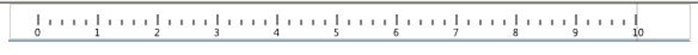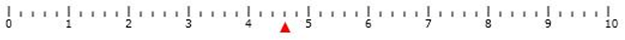# Canvas Control in Silverlight

Introduction

This article demonstrates how to create a scale on a canvas. We can create a scale on a canvas control in Silverlight. For that we have created a custom control named Ruler Control in Silverlight.

Use this control on a canvas in our Silverlight application.

Step 1: Create a Ruler Control in Silverlight.

In RulerControl.Xaml file we have a canvas named LayoutRoot.

<Canvas x:Name="LayoutRoot" Background="White" />

Draw a line with the help of function in Silverlight. So we can add those lines on the canvas.

In RulerControl.Xaml.cs, we have a method which draws the lines on the canvas.

public RulerControl()
{
InitializeComponent();
}

{
}

{
int count = 0;
for (int i = 0; i <= 500; i++)
{
if (i == 0 || i % 50 == 0)
{
Line l = new Line
{
Stroke = new SolidColorBrush(Colors.Black),
X1 = i,
Y1 = this.Height - 2,
X2 = i,
Y2 = this.Height - 2 - this.Height / 2
};

TextBlock tb = new TextBlock();
tb.Text = count.ToString();
tb.FontSize = 9;
tb.SetValue(Canvas.LeftProperty, (double)(i - 3));
tb.SetValue(Canvas.TopProperty, 15.5);
count++;

}

else if (i % 10 == 0)
{
Line l = new Line
{
Stroke = new SolidColorBrush(Colors.Black),
X1 = i,
Y1 = this.Height - 2,
X2 = i,
Y2 = this.Height - 2 - this.Height / 4
};
}

}
}

Step 2: Use a RulerControl on another Silverlight page

Let's say we have MainPage.Xaml.

<x:Class="SilverlightApplication.MainPage">
<xmlns:rulerctrl="clr-namespace:SilverlightApplication.Controls">

<Canvas Height="27"  Name="canvas1"  Width="522" Background="White" Canvas.Left="27" >
<rulerctrl:RulerControl Height="18" VerticalAlignment="Top"x:Name="firstruler"/>
</
Canvas>

It looks like as below.Step 3: Move button on canvas scale.

We can move the button on the canvas. Take one Button in the Canvas as follows.

<Grid x:Name="LayoutRoot" Background="White">
<Canvas Height="27"  Name="canvas1"  Width="522" Background="White" VerticalAlignment="Top" MouseMove="canvas1_MouseMove">
<rulerctrl:RulerControl Height="18" VerticalAlignment="Top" x:Name="firstruler"></rulerctrl:RulerControl>
<Button Height="18" Width="20" Canvas.Left="61" Canvas.Top="34" x:Name="btnFirst" Content="F" Style="{StaticResource btnstyle }">
<Button.RenderTransform>
<RotateTransform Angle="180"></RotateTransform>
</Button.RenderTransform>
</Button>
</Canvas>
</Grid>

Here we have MouseMove event of canvas. Using this we can move the button on canvas.
Like,

private void canvas1_MouseMove(object sender, MouseEventArgs e)
{
btnFirst.SetValue(Canvas.LeftProperty, e.GetPosition(canvas1).X);
}

Output looks like as followingSummary :

We can create a scale on a canvas; we can also move the button on the canvas.as ruler in word file.